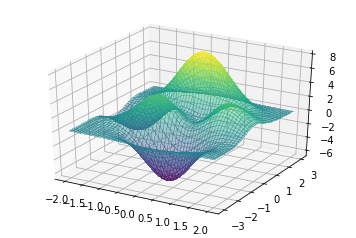Total: 25 pts

Start date: Friday Sept. 13
Due date: Tuesday Sept. 24

In this homework, you will learn to code one of the building block of many learning algorithms. When one wants to fit a model $f_{\beta}$ (with parameters $\beta$) to some training dataset $\mathcal{D}_T$, as we saw in regression, we usually solve an optimization problem of the form

\begin{align} \min_{f_{\beta}} \sum_{i\in \mathcal{D}_T} \left|f_{\beta}(x_i) - t_i\right|^2 \end{align}

When the learning model is relatively simple, such as in linear regression, an explicit solution can be computed in closed form as we saw this week. However, for most models (and particularly for the more interesting ones), such explicit expression does not exists or is not straightforward and we thus need an alternative.

The gradient descent algorithm takes any loss function $\ell(\beta, x)$ for which one wants to find a local minimum, i.e.

\begin{align} \min_{\beta} \ell(\beta; x, t) \end{align}

and iterates and the following step

\begin{align} \beta^{t+1} \leftarrow \beta^{t} - \alpha\; \text{grad}\; f(\beta^t) \end{align}

for a learning rate $\alpha$.

As a starting point, consider the following function whose plot is given below. You can uncomment the first line to activate interactive plot on jupyter and use the "plt.ioff() %matplotlib inline" cell below to turn the interactive mode off

$$f(x,y) = 3(1-x)^2 e^{-(x^2) - (y+1)^2}- 10(x/5 - x^3 - y^5)e^{-x^2-y^2}- \frac{1}{3} e^{-(x+1)^2 - y^2}$$
In :
#%matplotlib notebook # use to activate interactive plotting
import numpy as np

x = np.linspace(-2,2,100)
y = np.linspace(-3,3,100)

xx, yy = np.meshgrid(x, y, indexing='xy')

z = 3*((1-xx)**2) * np.exp(-(xx**2) - (yy+1)**2) \
- 10*(xx/5 - xx**3 - yy**5) * np.exp(-xx**2 - yy**2)- (1/3)* np.exp(-(xx+1)**2 - yy**2)

from matplotlib import cm

from mpl_toolkits.mplot3d import Axes3D
from mpl_toolkits.mplot3d import axes3d

fig = plt.figure()

ax.plot_surface(xx, yy, z, cmap=cm.viridis,alpha=.4,
linewidth=0, antialiased=False)

plt.show()1a.(10 pts) Code a function 'gradient_descent' that takes as argument a function 'f(x,y)' in two variables and a learning rate $\alpha$ as well as a maximum number of iterations $N$ and return the location $(x,y)$ of a local minimum as well as the value of the function and gradient after the $N$ iterations.

(Hint: note that you don't need to know the explicit expression for the derivative of f(x,y), you can simply use the definition of the derivative $$\frac{\partial f}{\partial y} \approx \frac{[f(x, y+h) - f(x,y)]}{h}$$ for a sufficiently small step size h and similarly for $x$.

In [ ]:
# First defining the function

def my_function(x,y):

z = 3*((1-x)**2) * np.exp(-(x**2) - (y+1)**2) \
- 10*(x/5 - x**3 - y**5) * np.exp(-x**2 - y**2)- (1/3)* np.exp(-(x+1)**2 - y**2)

return z



1b.(5pts) Extend your algorithm to a function of $D$ variables, $f(x_1, x_2, \ldots, x_D)$

In [ ]:
# put the code here


1b.(10 pts) Now that you have a gradient descent algorithm, we want to use it on a simple regression problem. Apply your GD iterations to the regression problem below.

• Generate and plot the line $t(x) = \beta_0 + \beta_1 x$ for $\beta_0 = 1$ and $\beta_1 = 2$. (use linspace with 100 points between $-5$ and $5$)

• Generate $20$ samples along the line (use linspace with 20). For each of those samples, generate the data/feature vectors $(t_i, x_i, x_i^2)$ where $t_i$ si defined as \begin{align} t_i = x_i + \varepsilon_i \end{align}

and $\varepsilon_i$ is some random gaussian noise with mean $0$ and variance $3$.

• Solve the minimization problem
\begin{align} \min_{\beta_0, \beta_1, \beta_2} \sum_{i=1}^{20}\left|t_i - \left(\beta_0 + \beta_1x_i + \beta_2 x_i^2\right)\right|^2 + \lambda \|\mathbf{\beta}\|_2^2 \end{align}

where $\mathbf{\beta} = (\beta_0, \beta_1,\beta_2)$, with your gradient descent algorithm.

• Repeat the steps above for a few values of $\lambda$. For each value of $\lambda$, apply the model you get on a new set of $20$ points between $-5$ and $5$ that you perturb with the same gaussian noise as before, i.e. $t_i = x_i + \varepsilon_i$, apply your model to those new points and compute the error
\begin{align} \text{err}(\lambda) = \frac{1}{20}\sum_{i=1}^{20} \left|t_i - \left(\beta_0 + \beta_1x_i +\beta_2 x_i^2 \right)\right|^2 \end{align}

Then plot the values $\text{err}(\lambda)$ as a function of $\lambda$.

In [ ]:
import numpy as np